•文档
• 今天小编就为大家分享一篇Pytorch实现神经网络的分类方式，具有很好的参考价值，希望对大家有所帮助。一起跟随小编过来看看吧
• 今天小编就为大家分享一篇pytorch-神经网络拟合曲线实例，具有很好的参考价值，希望对大家有所帮助。一起跟随小编过来看看吧
• ## PyTorch动态神经网络

千次阅读 2019-02-14 17:40:58
因为 PyTorch 是一个使用 PyTorch 语言的神经网络库, Torch 很好用, 但是 Lua 又不是特别流行, 所有开发团队将 Lua 的 Torch 移植到了更流行的语言 Python 上. 是的 PyTorch 一出生就引来了剧烈的反响. 为什么...
PyTorch 是 PyTorch 在 Python 上的衍生. 因为 PyTorch 是一个使用 PyTorch 语言的神经网络库, Torch 很好用, 但是 Lua 又不是特别流行, 所有开发团队将 Lua 的 Torch 移植到了更流行的语言 Python 上. 是的 PyTorch 一出生就引来了剧烈的反响. 为什么呢?
而且如果你知道 Numpy, PyTorch 说他就是在神经网络领域可以用来替换 numpy 的模块.
PyTorch 和 Tensorflow
据 PyTorch 自己介绍, 他们家的最大优点就是建立的神经网络是动态的, 对比静态的 Tensorflow, 他能更有效地处理一些问题, 比如说 RNN 变化时间长度的输出. 而我认为, 各家有各家的优势和劣势, 所以我们要以中立的态度. 两者都是大公司, Tensorflow 自己说自己在分布式训练上下了很大的功夫, 那我就默认 Tensorflow 在这一点上要超出 PyTorch, 但是 Tensorflow 的静态计算图使得他在 RNN 上有一点点被动 (虽然它用其他途径解决了), 不过用 PyTorch 的时候, 你会对这种动态的 RNN 有更好的理解.
而且 Tensorflow 的高度工业化, 它的底层代码… 你是看不懂的. PyTorch 好那么一点点, 如果你深入 API, 你至少能比看 Tensorflow 多看懂一点点 PyTorch 的底层在干嘛.
最后我的建议就是:
如果你是学生, 随便选一个学, 或者稍稍偏向 PyTorch, 因为写代码的时候应该更好理解. 懂了一个模块, 转换 Tensorflow 或者其他的模块都好说.如果是上班了, 跟着你公司来, 公司用什么, 你就用什么, 不要脱群.
PyTorch安装
学习资料:
PyTorch 官网
安装
PyTorch 安装起来很简单, 它自家网页上就有很方便的选择方式 (网页升级改版后可能和下图有点不同):
然后根据上面的提示, 我只需要在我的 Terminal 当中输入以下指令就好了:
$pip install http://download.pytorch.org/whl/torch-0.1.11.post5-cp35-cp35m-macosx_10_7_x86_64.whl$ pip install torchvision
Torch 或 Numpy
Torch 自称为神经网络界的 Numpy, 因为他能将 torch 产生的 tensor 放在 GPU 中加速运算 (前提是你有合适的 GPU), 就像 Numpy 会把 array 放在 CPU 中加速运算. 所以神经网络的话, 当然是用 Torch 的 tensor 形式数据最好咯. 就像 Tensorflow 当中的 tensor 一样.
当然, 我们对 Numpy 还是爱不释手的, 因为我们太习惯 numpy 的形式了. 不过 torch 看出来我们的喜爱, 他把 torch 做的和 numpy 能很好的兼容. 比如这样就能自由地转换 numpy array 和 torch tensor 了:
import torch
import numpy as np

np_data = np.arange(6).reshape((2, 3))
torch_data = torch.from_numpy(np_data)
tensor2array = torch_data.numpy()
print(
'\nnumpy array:', np_data,          # [[0 1 2], [3 4 5]]
'\ntorch tensor:', torch_data,      #  0  1  2 \n 3  4  5    [torch.LongTensor of size 2x3]
'\ntensor to array:', tensor2array, # [[0 1 2], [3 4 5]]
Torch 中的数学运算
其实 torch 中 tensor 的运算和 numpy array 的如出一辙, 我们就以对比的形式来看. 如果想了解 torch 中其它更多有用的运算符, API就是你要去的地方.
# abs 绝对值计算
data = [-1, -2, 1, 2]
tensor = torch.FloatTensor(data)  # 转换成32位浮点 tensor
print(
'\nabs',
'\nnumpy: ', np.abs(data),          # [1 2 1 2]
'\ntorch: ', torch.abs(tensor)      # [1 2 1 2]
)

# sin   三角函数 sin
print(
'\nsin',
'\nnumpy: ', np.sin(data),      # [-0.84147098 -0.90929743  0.84147098  0.90929743]
'\ntorch: ', torch.sin(tensor)  # [-0.8415 -0.9093  0.8415  0.9093]
)

# mean  均值
print(
'\nmean',
'\nnumpy: ', np.mean(data),         # 0.0
'\ntorch: ', torch.mean(tensor)     # 0.0
除了简单的计算, 矩阵运算才是神经网络中最重要的部分. 所以我们展示下矩阵的乘法. 注意一下包含了一个 numpy 中可行, 但是 torch 中不可行的方式.
# matrix multiplication 矩阵点乘
data = [[1,2], [3,4]]
tensor = torch.FloatTensor(data)  # 转换成32位浮点 tensor
# correct method
print(
'\nmatrix multiplication (matmul)',
'\nnumpy: ', np.matmul(data, data),     # [[7, 10], [15, 22]]
'\ntorch: ', torch.mm(tensor, tensor)   # [[7, 10], [15, 22]]
)

# !!!!  下面是错误的方法 !!!!
data = np.array(data)
print(
'\nmatrix multiplication (dot)',
'\nnumpy: ', data.dot(data),        # [[7, 10], [15, 22]] 在numpy 中可行
'\ntorch: ', tensor.dot(tensor)     # torch 会转换成 [1,2,3,4].dot([1,2,3,4) = 30.0
)

新版本中(>=0.3.0), 关于 tensor.dot() 有了新的改变, 它只能针对于一维的数组. 所以上面的有所改变.
tensor.dot(tensor)     # torch 会转换成 [1,2,3,4].dot([1,2,3,4) = 30.0

# 变为
torch.dot(tensor.dot(tensor)
变量
什么是 Variable
在 Torch 中的 Variable 就是一个存放会变化的值的地理位置. 里面的值会不停的变化. 就像一个裝鸡蛋的篮子, 鸡蛋数会不停变动. 那谁是里面的鸡蛋呢, 自然就是 Torch 的 Tensor 咯. 如果用一个 Variable 进行计算, 那返回的也是一个同类型的 Variable.
我们定义一个 Variable:
import torch
from torch.autograd import Variable # torch 中 Variable 模块

# 先生鸡蛋
tensor = torch.FloatTensor([[1,2],[3,4]])

print(tensor)
"""
1  2
3  4
[torch.FloatTensor of size 2x2]
"""

print(variable)
"""
Variable containing:
1  2
3  4
[torch.FloatTensor of size 2x2]
"""
Variable 计算, 梯度
我们再对比一下 tensor 的计算和 variable 的计算.
t_out = torch.mean(tensor*tensor)       # x^2
v_out = torch.mean(variable*variable)   # x^2
print(t_out)
print(v_out)    # 7.5
到目前为止, 我们看不出什么不同, 但是时刻记住, Variable 计算时, 它在背景幕布后面一步步默默地搭建着一个庞大的系统, 叫做计算图, computational graph. 这个图是用来干嘛的? 原来是将所有的计算步骤 (节点) 都连接起来, 最后进行误差反向传递的时候, 一次性将所有 variable 里面的修改幅度 (梯度) 都计算出来, 而 tensor 就没有这个能力啦.
v_out = torch.mean(variable*variable) 就是在计算图中添加的一个计算步骤, 计算误差反向传递的时候有他一份功劳, 我们就来举个例子:
v_out.backward()    # 模拟 v_out 的误差反向传递

# 下面两步看不懂没关系, 只要知道 Variable 是计算图的一部分, 可以用来传递误差就好.
# v_out = 1/4 * sum(variable*variable) 这是计算图中的 v_out 计算步骤
# 针对于 v_out 的梯度就是, d(v_out)/d(variable) = 1/4*2*variable = variable/2

'''
0.5000  1.0000
1.5000  2.0000
'''
获取 Variable 里面的数据 ¶
直接print(variable)只会输出 Variable 形式的数据, 在很多时候是用不了的(比如想要用 plt 画图), 所以我们要转换一下, 将它变成 tensor 形式.
print(variable)     #  Variable 形式
"""
Variable containing:
1  2
3  4
[torch.FloatTensor of size 2x2]
"""

print(variable.data)    # tensor 形式
"""
1  2
3  4
[torch.FloatTensor of size 2x2]
"""

print(variable.data.numpy())    # numpy 形式
"""
[[ 1.  2.]
[ 3.  4.]]
"""
激励函数
在具体的例子中, 我们默认首选的激励函数是哪些. 在少量层结构中, 我们可以尝试很多种不同的激励函数. 在卷积神经网络 Convolutional neural networks 的卷积层中, 推荐的激励函数是 relu. 在循环神经网络中 recurrent neural networks, 推荐的是 tanh 或者是 relu (这个具体怎么选, 我会在以后循环神经网络的介绍中在详细讲解).
一句话概括 Activation: 就是让神经网络可以描述非线性问题的步骤, 是神经网络变得更强大. 如果还不是特别了解, 我有制作一个动画短片, 浅显易懂的阐述了激励函数的作用. 包懂.
Torch 中的激励函数
Torch 中的激励函数有很多, 不过我们平时要用到的就这几个. relu, sigmoid, tanh, softplus. 那我们就看看他们各自长什么样啦.
import torch
import torch.nn.functional as F     # 激励函数都在这

# 做一些假数据来观看图像
x = torch.linspace(-5, 5, 200)  # x data (tensor), shape=(100, 1)
x = Variable(x)

接着就是做生成不同的激励函数数据:
x_np = x.data.numpy()   # 换成 numpy array, 出图时用

# 几种常用的 激励函数
y_relu = F.relu(x).data.numpy()
y_sigmoid = F.sigmoid(x).data.numpy()
y_tanh = F.tanh(x).data.numpy()
y_softplus = F.softplus(x).data.numpy()
# y_softmax = F.softmax(x)  softmax 比较特殊, 不能直接显示, 不过他是关于概率的, 用于分类

接着我们开始画图, 画图的代码也在下面: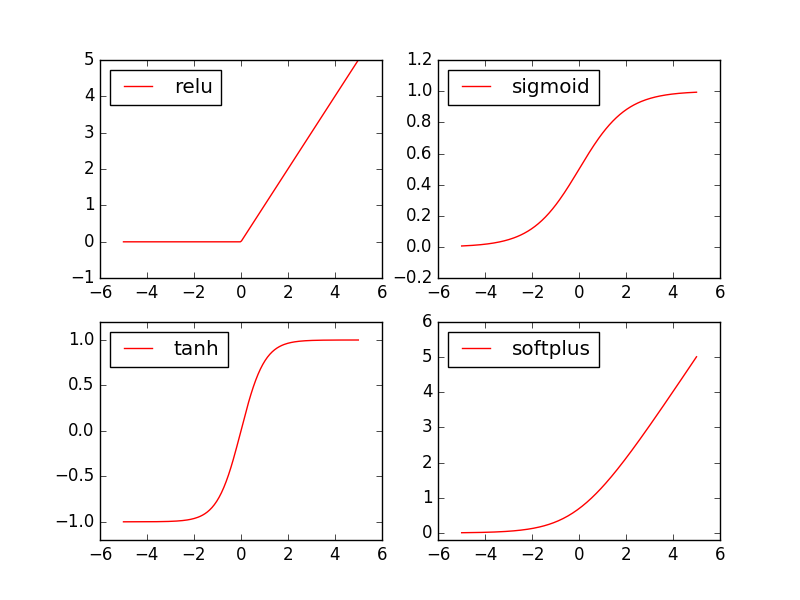import matplotlib.pyplot as plt  # python 的可视化模块, 我有教程 (https://morvanzhou.github.io/tutorials/data-manipulation/plt/)

plt.figure(1, figsize=(8, 6))
plt.subplot(221)
plt.plot(x_np, y_relu, c='red', label='relu')
plt.ylim((-1, 5))
plt.legend(loc='best')

plt.subplot(222)
plt.plot(x_np, y_sigmoid, c='red', label='sigmoid')
plt.ylim((-0.2, 1.2))
plt.legend(loc='best')

plt.subplot(223)
plt.plot(x_np, y_tanh, c='red', label='tanh')
plt.ylim((-1.2, 1.2))
plt.legend(loc='best')

plt.subplot(224)
plt.plot(x_np, y_softplus, c='red', label='softplus')
plt.ylim((-0.2, 6))
plt.legend(loc='best')

plt.show()

关系拟合（回归）

我会这次会来见证神经网络是如何通过简单的形式将一群数据用一条线条来表示. 或者说, 是如何在数据当中找到他们的关系, 然后用神经网络模型来建立一个可以代表他们关系的线条.
建立数据集
我们创建一些假数据来模拟真实的情况. 比如一个一元二次函数: y = a * x^2 + b, 我们给 y数据加上一点噪声来更加真实的展示它.
import torch
import matplotlib.pyplot as plt

x = torch.unsqueeze(torch.linspace(-1, 1, 100), dim=1)  # x data (tensor), shape=(100, 1)
y = x.pow(2) + 0.2*torch.rand(x.size())                 # noisy y data (tensor), shape=(100, 1)

# 画图
plt.scatter(x.data.numpy(), y.data.numpy())
plt.show()
建立神经网络
建立一个神经网络我们可以直接运用 torch 中的体系. 先定义所有的层属性(__init__()), 然后再一层层搭建(forward(x))层于层的关系链接. 建立关系的时候, 我们会用到激励函数, 如果还不清楚激励函数用途的同学, 这里有非常好的一篇动画教程.
import torch
import torch.nn.functional as F     # 激励函数都在这

class Net(torch.nn.Module):  # 继承 torch 的 Module
def __init__(self, n_feature, n_hidden, n_output):
super(Net, self).__init__()     # 继承 __init__ 功能
# 定义每层用什么样的形式
self.hidden = torch.nn.Linear(n_feature, n_hidden)   # 隐藏层线性输出
self.predict = torch.nn.Linear(n_hidden, n_output)   # 输出层线性输出

def forward(self, x):   # 这同时也是 Module 中的 forward 功能
# 正向传播输入值, 神经网络分析出输出值
x = F.relu(self.hidden(x))      # 激励函数(隐藏层的线性值)
x = self.predict(x)             # 输出值
return x

net = Net(n_feature=1, n_hidden=10, n_output=1)

print(net)  # net 的结构
"""
Net (
(hidden): Linear (1 -> 10)
(predict): Linear (10 -> 1)
)
"""
训练网络
训练的步骤很简单, 如下:
# optimizer 是训练的工具
optimizer = torch.optim.SGD(net.parameters(), lr=0.2)  # 传入 net 的所有参数, 学习率
loss_func = torch.nn.MSELoss()      # 预测值和真实值的误差计算公式 (均方差)

for t in range(100):
prediction = net(x)     # 喂给 net 训练数据 x, 输出预测值

loss = loss_func(prediction, y)     # 计算两者的误差

loss.backward()         # 误差反向传播, 计算参数更新值
optimizer.step()        # 将参数更新值施加到 net 的 parameters 上
可视化训练过程
为了可视化整个训练的过程, 更好的理解是如何训练, 我们如下操作:
import matplotlib.pyplot as plt

plt.ion()   # 画图
plt.show()

for t in range(200):

...
loss.backward()
optimizer.step()

# 接着上面来
if t % 5 == 0:
# plot and show learning process
plt.cla()
plt.scatter(x.data.numpy(), y.data.numpy())
plt.plot(x.data.numpy(), prediction.data.numpy(), 'r-', lw=5)
plt.text(0.5, 0, 'Loss=%.4f' % loss.data.numpy(), fontdict={'size': 20, 'color':  'red'})
plt.pause(0.1)

展开全文• Pytorch卷积神经网络GPU加速 首先要配置好pytorch，通常我们直接pip install pytorch的话会缺少关于cude的内容，可以通过torch.cuda.is_available()来判断是否需要重新安装pytorch 如果返回False 我们需要到官方...
Pytorch卷积神经网络GPU加速
首先要配置好pytorch，通常我们直接pip install pytorch的话会缺少关于cuda的内容，可以通过torch.cuda.is_available()来判断是否需要重新安装pytorch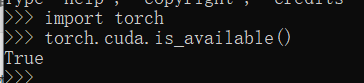如果返回False 我们需要到官方重新下载pytorch（https://pytorch.org/），在此之前我们可以pip uninstall 来卸载已有的torch包。 1.官方找到对应自己电脑版本的包 首先查看自己电脑cude版本，打开英伟达控制面板—帮助—系统信息—组件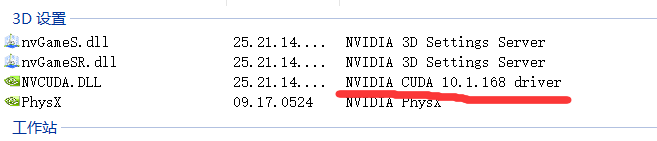我这里是10.1版本
然后查看过去版本，找到符合自己版本的包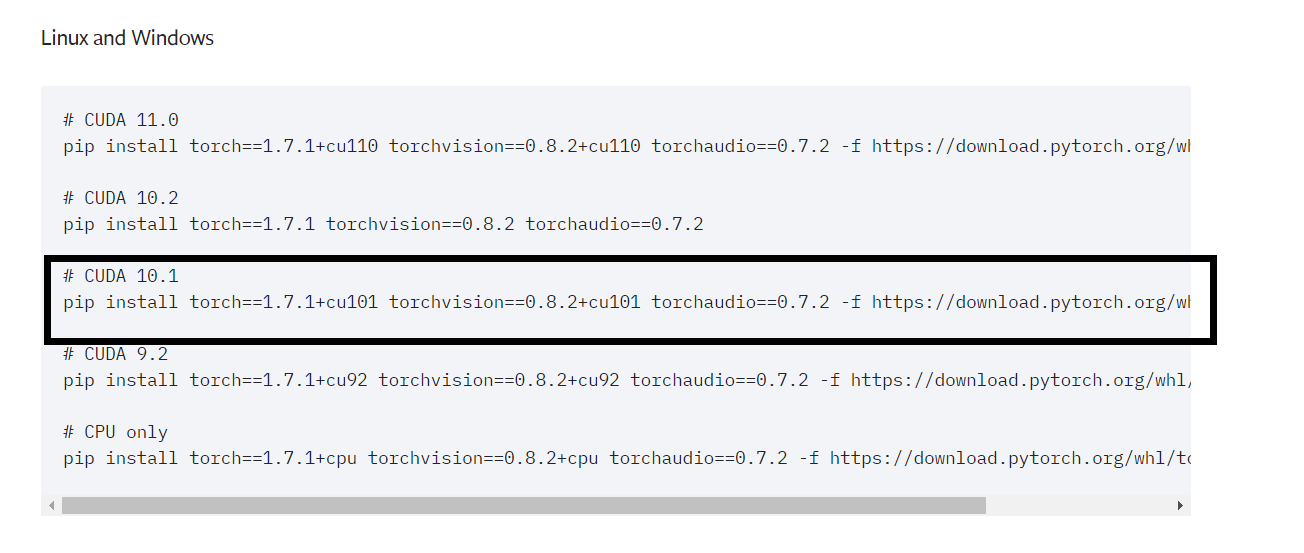这里我选择使用pip的命令，其中也有Conda的下载命令
tips: （1）网址是外网且文件有1G多比较大，需要搭梯子 （2）事先更新一下自己的pip（python -m pip install --upgrade pip），不然会下载到一半提示pip版本不够。 （3）我出现了很莫名其妙的超时情况，所以建议加一个default timeout pip --default-timeout = 一个时间例如10000 + install ~~~~~~
3.下载完成之后再次检验torch.cuda.is_available()，如果返回True则证明成功了
接下来这个博客有GPU加速CNN的具体讲解： https://blog.csdn.net/weixin_43826681/article/details/109776079
tips： （1）它的代码里会下载MNIST数据，源网址也是外网，建议搭梯子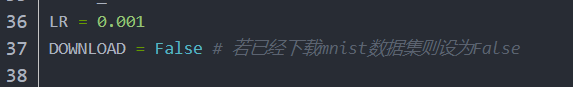第一次运行需要把它改成True （2）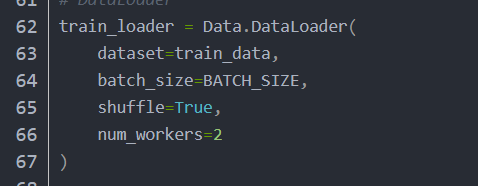他的代码这里的num_workers要改成0，这可以理解为多线程读取数据，windows好像不太支持，Linux才支持 （3）因为我们这次下载的pytorch版本比较新所以会出现报错
test_x = Variable(
torch.unsqueeze(test_data.data, dim=1),
volatile=True
).type(torch.FloatTensor)[:2000]/255 # 将将0~255压缩为0~1

上面的代码要改成下面的代码：
with torch.no_grad():
test_x = Variable(torch.unsqueeze(test_data.data, dim=1)).type(torch.FloatTensor)[:2000]/255 # 将将0~255压缩为0~1

展开全文机器学习
• #4.1_CNN_卷积神经网络_(PyTorch_tutorial_神经网络_教学)学习资源
• 使用PyTorch了解神经网络的基本知识 （工作正在进行中） 这是一系列文章，旨在了解作为神经网络的巫术（使用PyTorch）。 基本的 中间的
• #4.2_RNN_循环神经网络_分类_(PyTorch_tutorial_神经网络_教学)学习资源
• #4.3_RNN_循环神经网络_回归_(PyTorch_tutorial_神经网络_教学)学习资源
• 用NumPy实现两层神经网络 一个全连接ReLU神经网络，一个隐藏层，没有bias。用来从x预测y，使用Square Loss。 这一实现完全使用NumPy来计算前向神经网络，loss，和反向传播算法。 N—样本数据的大小 DinD_{in}Din​...
• 3.5 PyTorch神经网络的批数据训练.pdf
• 本博客一个小例子，使用 pytorch 来编写一个神经网络来拟合 sin 函数 废话少说，直接上代码： from torch.utils.data import DataLoader from torch.utils.data import TensorDataset import torch.nn as nn ...
• 使用pytorch搭建神经网络下载相关包直接上代码 下载相关包 其实就一个包，先下载这个软件 然后： 再等一会后apply 然后就可以直接import torch包了，如果有问题可以点击这个链接：链接: ...


使用pytorch搭建神经网络
下载相关包直接上代码

下载相关包
其实就一个包，先下载这个软件然后：再等一会后apply然后就可以直接import torch包了，如果有问题可以点击这个链接：链接: https://blog.csdn.net/qq_34403736/article/details/84726504.
直接上代码
import torch
import numpy as np
import os
os.environ["KMP_DUPLICATE_LIB_OK"]="TRUE"   #网上搜的解决防止报错的方案

"""
读取数据
"""
#读取数据函数
my_matrix = np.loadtxt(data_path ,dtype = float, usecols = , unpack = True)
return my_matrix

sum_matirx = np.zeros((1600,1))  #该数据有1600行
path = "E:\学校的一些资料\文档\大二暑假\数据\RamanSystem-master\RamanSystem-master\光谱(1)"#文件夹目录
files= os.listdir(path) #得到文件夹下的所有文件名称
txts = []
for file in files: #遍历文件夹
#print("file: ",file)
if file == "三文鱼" or file == "鲷鱼":
position = path+'\\'+ file #构造绝对路径，"\\"，其中一个'\'为转义符
files2 = os.listdir(position)
for file2 in files2:
#print("file2: ",file2)
position2 = position + '\\' + file2
sum_matirx = np.delete(sum_matirx,0,1)#删掉第一列，第一个数字代表删除的行或列号，第二个数字1代表删列，0代表删行
sum_matirx = sum_matirx.T
print("最初始的数据shape：\n",sum_matirx.shape)
#print("原始数据：\n",sum_matirx)

"""
PCA降维(引用库中的）
"""
import sklearn
from sklearn.decomposition import PCA#加载PCA算法包
dimension = int(4)#确定降维后的维度
category = int(2)#分类的标签数
pca = PCA(dimension)   #保留所有成分  #选取累计贡献率大于80%的主成分（dimension个主成分）
pca.fit(sum_matirx)
#print(pca.components_) #返回模型的各个特征向量
#print(pca.explained_variance_ratio_) #返回各个成分各自的方差百分比(也称贡献率）
low_d = pca.transform(sum_matirx)
low_d = torch.tensor(low_d, dtype=torch.float32)
#print("low_d: \n",low_d)
print("low_d.shape: \n", low_d.shape)

"""
设置标签
"""
type_num = 50 #每组样本数
low_d_labels = torch.zeros(low_d.shape, dtype=torch.float32)
for i in range(category):
for j in range(type_num):
low_d_labels[i*type_num + j] = (int)(i+1)
#print("low_d_labels: \n", low_d_labels)
print("low_d_labels.shape: \n", low_d_labels.shape)

"""
数据处理分类
low_d 降维后的特征值
low_d_labels 对应的标签（1~8）
"""
test_num = 10 #测试样例数
x_train = low_d[0:type_num-test_num]
y_train = low_d_labels[0:type_num-test_num]
for i in range(1,category):
index = i*type_num
x_train = torch.cat([x_train,low_d[index:index + type_num-test_num]], 0)  #第二个参数是指在哪个维度进行拼接
y_train = torch.cat([y_train,low_d_labels[index:index + type_num-test_num]], 0)
#把测试样本拼接到后面40个
for i in range(0, category):
index = i*type_num
x_train = torch.cat([x_train,low_d[index+type_num-test_num:index + type_num]], 0)
y_train = torch.cat([y_train,low_d_labels[index+type_num-test_num:index + type_num]], 0)
print("x_train.shape: \n",x_train.shape)
print("y_train.shape: \n",y_train.shape)

"""
书上代码
"""
#确定网络结构
#三层神经网络，前2个隐藏层分别设置为6个和2个神经元，并使用逻辑函数激活，最后1层输出层有1个神经元
import torch.nn as nn
hidden_features = [240, 8]  #前2个隐藏层分别设置为6个和2个神经元
layers = [nn.Linear(4, hidden_features), ]
for idx, hidden_feature in enumerate(hidden_features):
layers.append(nn.Tanh())
next_hidden_feature = hidden_features[idx + 1] \
if idx + 1 < len(hidden_features) else 1   #这个1是指组后的输出层的维度
layers.append(nn.Linear(hidden_feature, next_hidden_feature))
net = nn.Sequential(*layers)  #前馈神经网络
print('神经网络为: \n',format(net))

#训练
import torch.optim
criterion = nn.MSELoss()
#将数据转化为张量
features = x_train
labels = y_train
print("features.shape: \n", features.shape)
print("labels.shape: \n", labels.shape)

train_entry_num = 80 #选择训练样本数
train_num = 20 #选择测试样本数

n_iter = 100000 #最大迭代次数
for step in range(n_iter):
outputs = net(features)
preds = outputs.squeeze() #默认去掉维数为1的维度，就是少掉一层中括号
#print("preds.shape: \n",preds.shape)

loss_train = criterion(preds[:train_entry_num], labels[:train_entry_num])

loss_validate = criterion(preds[train_entry_num:train_entry_num + train_num], labels[train_entry_num:train_entry_num + train_num])

if step % 10000 == 0:
print('#{} 训练集MSE = {:g}, 验证集MSE = {:g}'.format(step, loss_train, loss_validate))
#print("验证集的大小：\n",preds[train_entry_num:train_entry_num + train_num].shape)
print("验证集的预测值：\n",preds[train_entry_num:train_entry_num + train_num])
print("验证集的标准值：\n",labels[train_entry_num:train_entry_num + train_num])

loss_train.backward()
optimizer.step()
#print("outputs: \n", outputs)
count = 0
for i in range (train_num):
temp = preds[i]
if temp >= 0.5 and temp < 1.5:
temp = 1
if temp >= 1.5 and temp < 2.5:
temp = 2
if temp == labels[i]:
count = count + 1
print("预测准确数：\n",count)
print("预测准确率：\n",count / train_num)
print ('训练集MSE = {:g}, 验证集MSE = {:g}'.format(loss_train, loss_validate))


声明：这些代码的主要思想都是我从一本书上弄下来的。
展开全文python 机器学习
• 最近发现身边的一些初学者朋友捧着各种pytorch指南一边看一边敲代码，到最后反而变成了打字员。 敲完代码一运行，出来结果和书上一对比，哦，是书上的结果，就翻到下一章。半天就能把一本书都打完，但是合上书好像...
最近发现身边的一些初学者朋友捧着各种pytorch指南一边看一边敲代码，到最后反而变成了打字员。
敲完代码一运行，出来结果和书上一对比，哦，是书上的结果，就翻到下一章。半天就能把一本书都打完，但是合上书好像什么都不记得。有的甚至看了两三遍，都搭不出一个简单的网络来，这种学习方式很不可取。如果你刚好是这种情况，这篇文章应该能给你一些帮助。如果你已经是进阶的水平了，就直接关掉页面就好了。
pytorch的网络搭建，比tensorflow简单很多。格式很好理解。

如果你想做一个网络，需要先定义一个Class，继承 nn.Module（这个是必须的,所以先import torch.nn as nn，nn是一个工具箱，很好用)，我们把class的名字就叫成Net.
Class Net (nn.Module):
这个Class里面主要写两个函数，一个是初始化的__init__函数，另一个是forward函数。我们随便搭一个，如下：
    def __init__(self):
super().__init__()
self.conv1=nn.Conv2d(1,6,5)
self.conv2=nn.Conv2d(6,16,5)

def forward(self, x):
x=F.max_pool2d(F.relu(self.conv1(x)),2)
x=F.max_pool2d(F.relu(self.conv2(x)),2)
return x
__init__里面就是定义卷积层，当然先得super()一下，给父类nn.Module初始化一下。(Python的基础知识）在这个里面主要就是定义卷积层的，比如第一层，我们叫它conv1，把它定义成输入1通道，输出6通道，卷积核5*5的的一个卷积层。conv2同理。神经网络“深度学习”其实主要就是学习卷积核里的参数，像别的不需要学习和改变的，就不用放进去。比如激活函数relu()，你非要放进去也行，再给它起个名字叫myrelu，也是可以的。
forward里面就是真正执行数据的流动。比如上面的代码，输入的x先经过定义的conv1（这个名字是你自己起的），再经过激活函数F.relu()（这个就不是自己起的名字了，最开始应该先import torch.nn.functional as F，F.relu()是官方提供的函数。当然如果你在__init__里面把relu定义成了我上面说的myrelu，那你这里直接第一句话就成了x=F.max_pool2d(myrelu(self.conv1(x)),2)。下一步的F.max_pool2d池化也是一样的，不多废话了。在一系列流动以后，最后把x返回到外面去。
这个Net的Class定义主要要注意两点。
第一：是注意前后输出通道和输入通道的一致性。不能第一个卷积层输出4通道，第二个输入6通道，这样就会报错。
第二：它和我们常规的python的class还有一些不同，发现了没有？我们该怎么用这个Net呢？
先定义一个Net的实例（毕竟Net只是一个类不能直接传参数，output=Net（input）当然不行）
net=Net()
这样我们就可以往里传x了，假设你已经有一个要往神经网络的输入的数据“input"（这个input应该定义成tensor类型，怎么定义tensor那就自己去看看书了。另外pytorch三点几版本的你还得把它弄成Variable类型，4.0以后的就不需要了）
在传入的时候，是:
output=net(input)
看之前的定义：
def __init__(self):
……

def forward(self, x):
……
有点奇怪。好像常规python一般向class里面传入一个数据x，在class的定义里面，应该是把这个x作为形参传入__init__函数里的，而在上面的定义中，x作为形参是传入forward函数里面的。
其实也不矛盾，因为你定义net的时候，是net=Net()，并没有往里面传入参数。如果你想初始化的时候按需传入，就把需要的传入进去。只是x是神经网络的输入，但是并非是初始化需要的，初始化一个网络，必须要有输入数据吗？未必吧。只是在传入网络的时候，会自动认为你这个x是喂给forward里面的。

在网络定义好以后，就涉及到传入参数，算误差，反向传播，更新权重…确实很容易记不住这些东西的格式和顺序。
传入的方式上面已经介绍了，相当于一次正向传播，把一路上各层的输入x都算出来了。
想让神经网络输出的output跟你期望的ground truth差不多，那就是不断减小二者间的差异，这个差异是你自己定义的，也就是目标函数（object function）或者就是损失函数。如果损失函数loss趋近于0，那么自然就达到目的了。
损失函数loss基本上没法达到0，但是希望能让它达到最小值，那么就是希望它能按照梯度进行下降。梯度下降的公式，大家应该都很熟悉，不熟悉的话，建议去看一下相关的理论。谁喜欢看公式呢？所以我这里不讲。
只是你的输入是由你来决定的，那神经网络能学习和决定什么呢？自然它只能决定每一层卷积层的权重。所以神经网络只能不停修改权重，比如y=wx+b，x是你给的，它只能改变w，b让最后的输出y尽可能接近你希望的y值，这样损失loss就越来越小。
如果loss对于卷积层里面的参数W的偏导数接近0了，不就意味着到达了一个极小值吗？而l在你的loss计算方式已经给定的情况下，loss对于w的偏导数的减小，其实只能通过更新参数卷积层参数W来实现（别的它决定不了啊，都是你输入和提供的）。
所以，通过下述方式实现对W的更新：（注意这些编号，下面还要提）
【1】 先算loss对于输入x的偏导，（当然网络好几层，这个x指的是每一层的输入，而不是最开始的输入input）
【2】 对【1】的结果再乘以一个步长（这样就相当于是得到一个对参数W的修改量）
【3】 用W减掉这个修改量，完成一次对参数W的修改。
说的不太严谨，但是大致意思是这样的。这个过程你可以手动实现，但是大规模神经网络怎么手动实现？那是不可能的事情。所以我们要利用框架pytorch和工具箱torch.nn。

所以要定义损失函数，以MSEloss为例：
compute_loss=nn.MSELoss()
明显它也是个类，不能直接传入输入数据，所以直接loss=nn.MSEloss（target，output）是不对的。需要把这个函数赋一个实例，叫成compute_loss。之后就可以把你的神经网络的输出，和标准答案target传入进去：
loss=compute_loss(target,output)
算出loss，下一步就是反向传播：
loss.backward()
这一步其实就是把【1】给算完了，得到对参数W一步的更新量，算是一次反向传播。
这里就注意了，loss.backward()是啥玩意？如果是自己的定义的loss（比如你就自己定义了个def loss（x，y）：return y-x ）这样肯定直接backward会出错。所以应当用nn里面提供的函数。当然搞深度学习不可能只用官方提供的loss函数，所以如果你要想用自己的loss函数，必须也把loss定义成上面Net的样子（不然你的loss不能反向传播，这点要注意，注：这点是以前写的，很久之前的版本不行，现在都可以了，基本不太需要这样了），也是继承nn.Module，把传入的参数放进forward里面，具体的loss在forward里面算，最后return loss。__init__()就空着，写个super().__init__就行了。
在反向传播之后，第【2】和第【3】怎么实现？就是通过优化器来实现。让优化器来自动实现对网络权重W的更新。
所以在Net定义完以后，需要写一个优化器的定义（选SGD方式为例）：
from torch import optim
optimizer=optim.SGD(net.parameters(),lr=0.001,momentum=0.9)
同样，优化器也是一个类，先定义一个实例optimizer，然后之后会用。注意在optimizer定义的时候，需要给SGD传入了net的参数parameters，这样之后优化器就掌握了对网络参数的控制权，就能够对它进行修改了。传入的时候把学习率lr也传入了。
在每次迭代之前，先把optimizer里存的梯度清零一下（因为W已经更新过的“更新量”下一次就不需要用了）
optimizer.zero_grad()
在loss.backward()反向传播以后，更新参数：
optimizer.step()
所以我们的顺序是：
1.先定义网络:写网络Net的Class，声明网络的实例net=Net()，
2.定义优化器optimizer=optim.xxx(net.parameters()，lr=xxx)，
3.再定义损失函数（自己写class或者直接用官方的，compute_loss=nn.MSELoss()或者其他。
4.在定义完之后，开始一次一次的循环：
②再将input传入，output=net(input) ，正向传播
③算损失，loss=compute_loss(target,output)   ##这里target就是参考标准值GT，需要自己准备，和之前传入的input一一对应
④误差反向传播，loss.backward()
⑤更新参数，optimizer.step()
这样就实现了一个基本的神经网络。大部分神经网络的训练都可以简化为这个过程，无非是传入的内容复杂，网络定义复杂，损失函数复杂，等等等等。
说的有问题的地方感谢指正。


展开全文快速入门
• ## PyTorch 卷积神经网络简介

千次阅读 多人点赞 2021-03-02 11:24:35
卷积神经网络简介概述架构卷积是什么图像颜色通道特征图个数堆叠的卷积层 概述 卷积神经网络在 CV 领域, 检测任务领域, 分类与检索领域, 图片重构领域, 医学任务领域, 无人驾驶领域, 人脸识别领域等等都有广泛的应用...
• 3.4 PyTorch神经网络的保存和提取方法.pdf
• 47_pytorch循环神经网络（学习笔记）.pdf
• 主要介绍了使用 pytorch 创建神经网络拟合sin函数的实现，文中通过示例代码介绍的非常详细，对大家的学习或者工作具有一定的参考学习价值，需要的朋友们下面随着小编来一起学习学习吧
• Pytorch 自定义神经网络训练自己的图片数据 利用pytorch来设计自定义的神经网络，准备自定义的数据集，训练分类自己的图片数据 本文利用pytorch训练一个自定义的分类器，来对自己的图片数据集进行分类。神经网络的...机器学习 自定义数据集
• 详情参考https://github.com/LianHaiMiao/pytorch-lesson-zh/，这个老师讲的特别详细，或者参考https://blog.csdn.net/a1103688841/article/details/89222614
• 这里有一个英文示例：https://towardsdatascience.com/how-to-code-a-simple-neural-network-in-pytorch-for-absolute-beginners-8f5209c50fdd，我觉得不错就搬了过来，代码中有一些问题，我修正了。 需要的头文件 ...深度学习 tensorflow hash 人工智能
• 比DGL快14倍：PyTorch神经网络库PyG上线了 图神经网络是最近 AI 领域最热门的方向之一，很多图神经网络框架如graph_nets和DGL已经上线。但看起来这些工具还有很多可以改进的空间。近日，来自德国多特蒙德工业大学...
• 在本文章中，我们将使用PyTorch从头开始实现一个简单的神经网络。 注：阅读本文之前，最好可以了解神经网络的工作原理。 虽然有很多库可以用于深度学习，但我最喜欢 PyTorch。作为一名python 程序员，我喜欢 PyTorch...程序人生 经验分享
• 打印查看pytorch神经网络模型及参数 net = resnet（）#实例化网络 for parameters in net.parameters():#打印出参数矩阵及值 print(parameters) for name, parameters in net.named_parameters():#打印出每一层...深度学习
• 这篇文章我们一起学习一下如何使用PyTorch搭建神经网络训练分类模型，这里我们用的数据集是Yann LeCun的MINIST数据集。首先我们来看看需要哪些python库： import torch import torch.nn as nn import torch.nn....分类
• 一旦您精通PyTorch语法并能够构建单层神经网络，您将通过配置和训练卷积神经网络（CNN）进行图像分类，逐步学习解决更复杂的数据问题。 在阅读本章的过程中，您将发现如何通过实现递归神经网络（RNN）解决NLP问题。...
• #1.2_安装_(PyTorch_tutorial_神经网络_教学)学习资源...